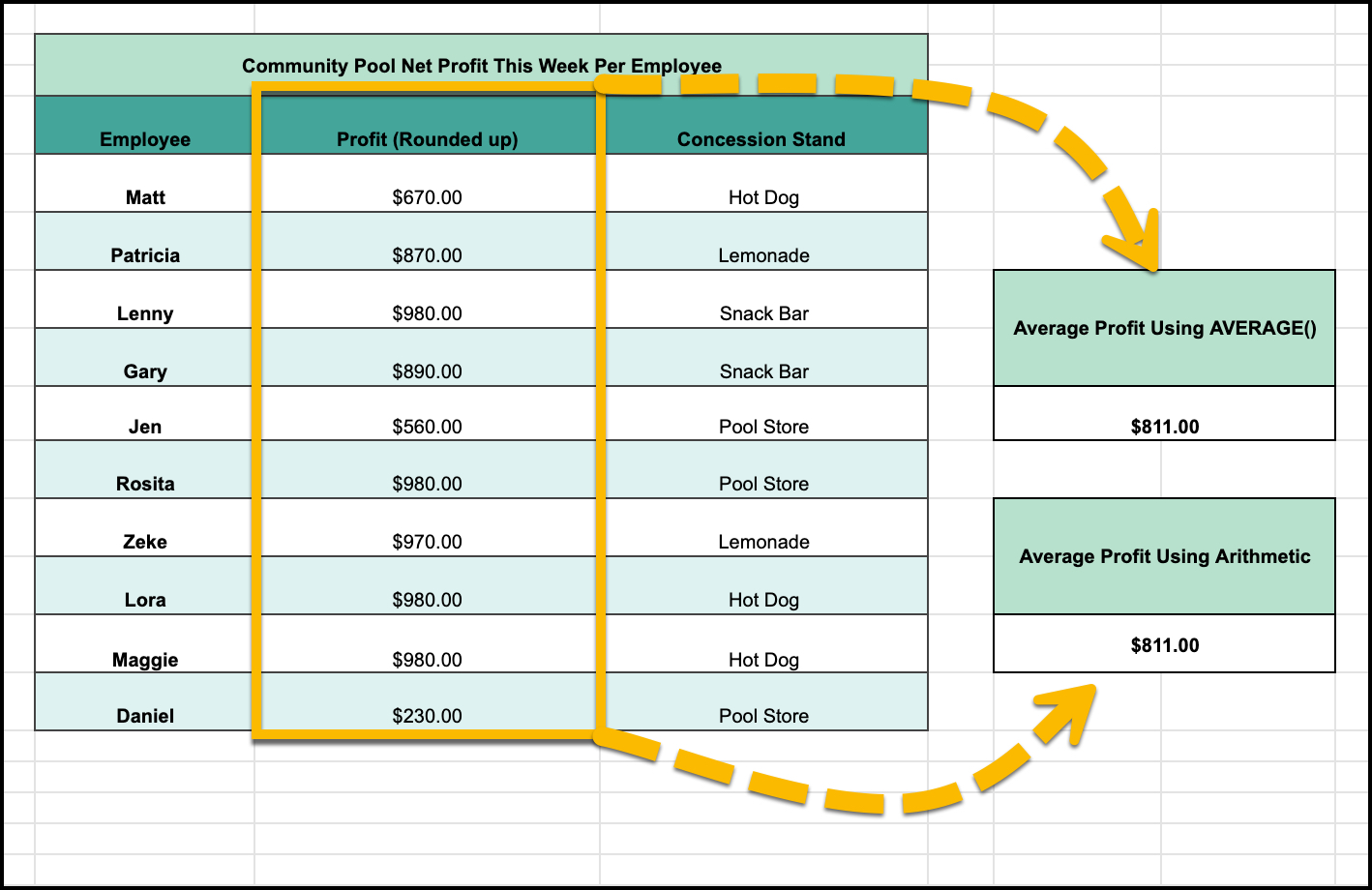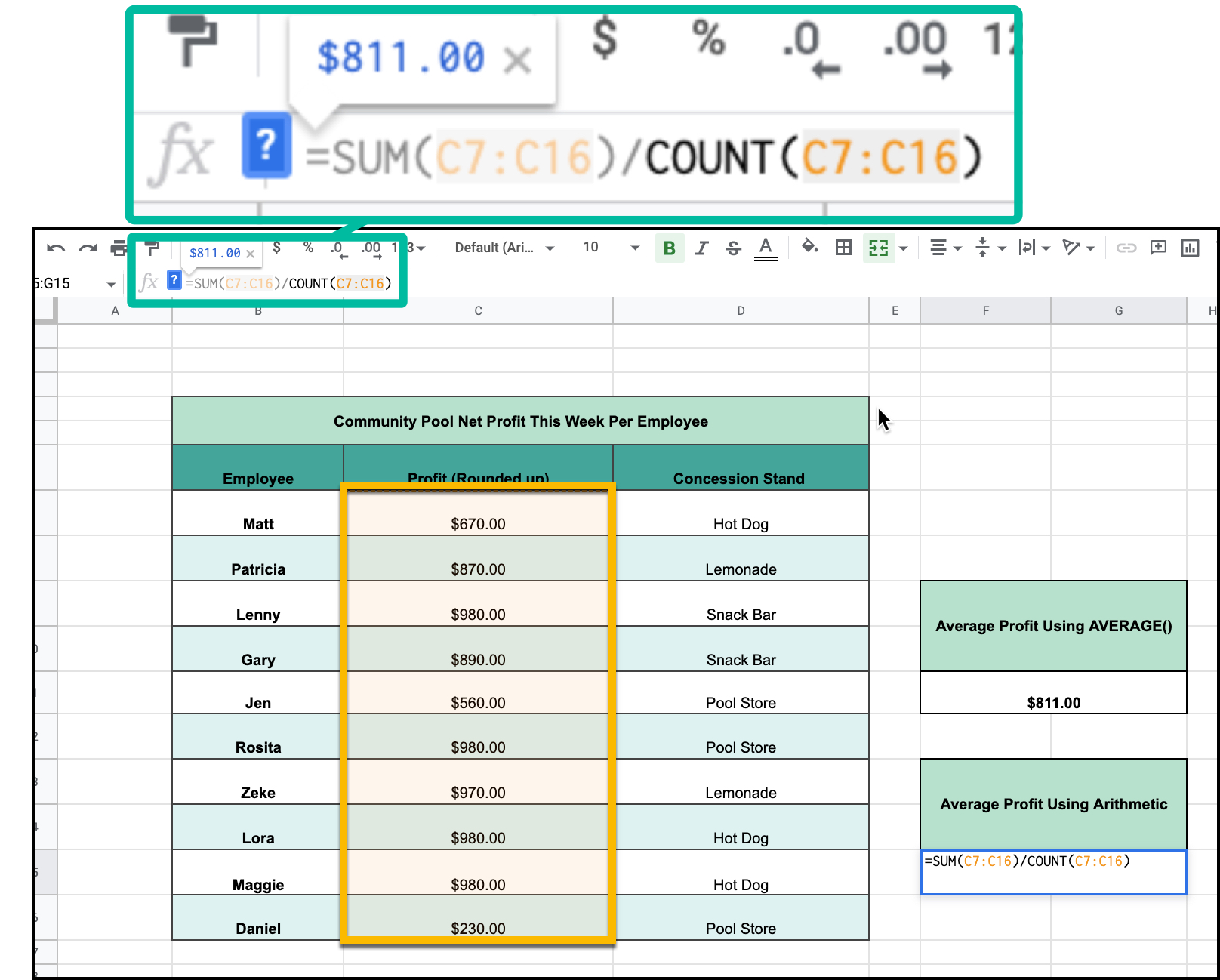# How to Average Numbers in Google sheets

Google Sheets is largely a tool used for managing statistics; what bigger statistic than averages? Learn how to calculate it here! There are two ways that you can calculate average in google sheets:

• Basic Arithmetic## =AVERAGE(Cell Range)

This is a very simple and straightforward technique when calculating the average in Google Sheets, which also factors into calculating standard deviation.. You can see a basic example in the image below.Simply click on the function window just above the column label row and type in

=AVERAGE() followed by the range of cells whose value you want to average inside the parentheses. That can be entered either manually or by clicking and dragging the desired cell range.

## =SUM(Cell Range)/COUNT(Cell Range)

Another way you can calculate the average of a cell range is by simply doing the bare bones arithmetic. In case you don't know, Average equals the sum of the data divided by the number of data values, also called count.

That can be achieved in Google Sheets using SUM() and COUNT() as shown below.And there you have it, that is how you can calculate the average of any cell range within google sheets!